Get instant live expert help with Excel or Google Sheets“My Excelchat expert helped me in less than 20 minutes, saving me what would have been 5 hours of work!”

#### Post your problem and you'll get expert help in seconds

Your message must be at least 40 characters
Our professional experts are available now. Your privacy is guaranteed.

# Get day name from date

Any date in Excel can be converted to its corresponding day name by customizing the display, or by using the TEXT, CHOOSE and WEEKDAY functions.  This step by step tutorial will assist all levels of Excel users in getting day name from date using three different methods.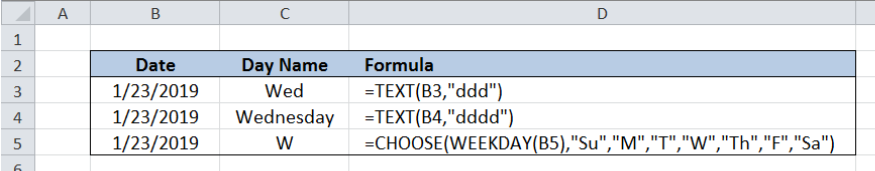Figure 1.  Final result:  Get day name from date

Formula 1: `=TEXT(B3,"ddd")`

Formula 2: `=TEXT(B4,"dddd")`

Formula 3: `=CHOOSE(WEEKDAY(B5),"Su","M","T","W","Th","F","Sa")`

## Syntax of TEXT Function

TEXT converts a number into text

`=TEXT(value, format_text)`

• value – any numeric value, a formula or cell reference to a numeric value
• format_text –  a numeric format expressed as a text string and enclosed in quotation marks
• “ddd” displays the day as an abbreviation from Sun to Sat
• “dddd” displays the day as a full name from Sunday to Saturday

## Syntax of CHOOSE Function

CHOOSE function returns a value from a list of values based on the index_num provided

`=CHOOSE(index_num, value1, [value2], ...)`

• index_num  – determines which value in the list of values is returned by the CHOOSE function
• Index_num must be a number between 1 and 254, or a cell reference with values between 1 and 254
• Value1 is returned if index_num is 1, values2 if index_num is 2; and so on
• CHOOSE returns the error #VALUE! if index_num is less than 1 or greater than the number of the last value in the list
• value1, value2, …   the values in the list  that the function chooses from; Only value 1 is required, succeeding values are optional

## Syntax of WEEKDAY Function

WEEKDAY returns the day of the week of the given date; returns an integer ranging from 1 to 7, where by default, 1 means Sunday and 7 means Saturday

`=WEEKDAY(serial_number,[return_type])`

• serial_number  – the date for which we want to find the corresponding day of the week
• return_type  – Optional; determines the type of return value; see below table for the options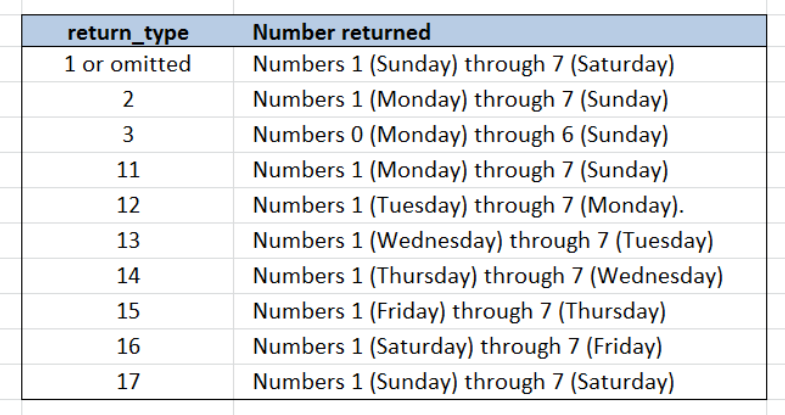Figure 2.  WEEKDAY function return_type options

## Setting up our Data

Our table contains two columns: Date (column B) and Day Name (column C).  We want to determine the name of the day corresponding to the date “1/23/2019” by using three different methods.  The results will be recorded in column D.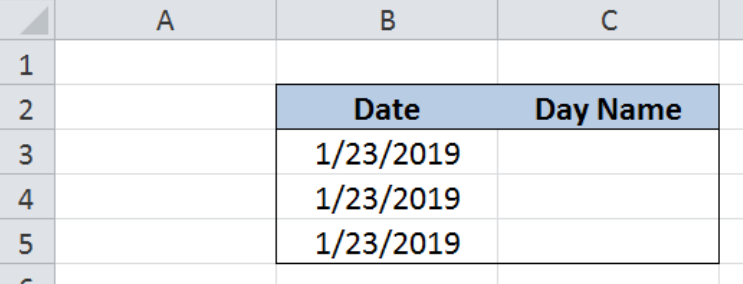Figure 3.  Sample data to get day name from date

## Get day name from date using TEXT function

One way to get the day name from a date is by using the TEXT function.  This method converts the date into a text string representing the day name of the given date.   Let us follow these steps:

Step 1.  Select cell C3

Step 2.  Enter the formula: `=``TEXT(B3,"ddd")`

Step 3.  Select cell C4

Step 4.  Enter the formula:`=``TEXT(B4,"dddd")`

Step 5.  Press ENTER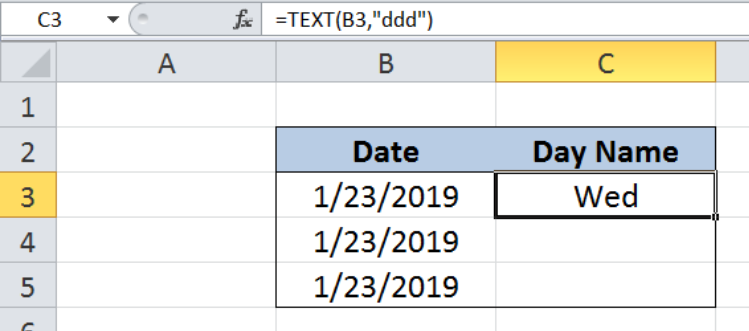Figure 4.  Entering the formula to get day name  in “ddd” format using TEXT

The formulas in C3 and C4 both use the TEXT function but with different format.  Our date, “1/23/2019” falls on a Wednesday.

In cell C3, the day of the week is represented as an abbreviation in three letters, “Wed”, which corresponds to the format “ddd”.  On the other hand, C4 returns the day of the week in full name through the use of the format”dddd”. Hence, the value in C4 is “Wednesday”.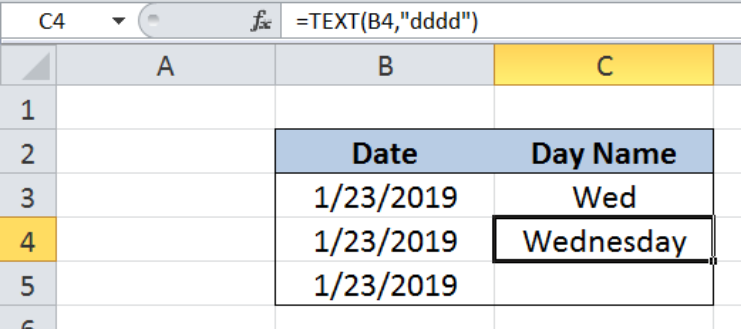Figure 5. TEXT function returns day name  in “dddd” format

## Get day name from date using CHOOSE and WEEKDAY functions

Another way to get the day name from a date is by using a combination of the CHOOSE and WEEKDAY functions.  Through this method, we can customize the day name that we want to show. Let us follow these steps:

Step 1.  Select cell C5

Step 2.  Enter the formula: `=``CHOOSE(WEEKDAY(B5),"Su","M","T","W","Th","F","Sa")`

Step 3.  Press ENTER

In this example, we customize the day name by setting up the values in the list within our formula:  “Su“,”M“,”T“,”W“,”Th“,”F“,”Sa“.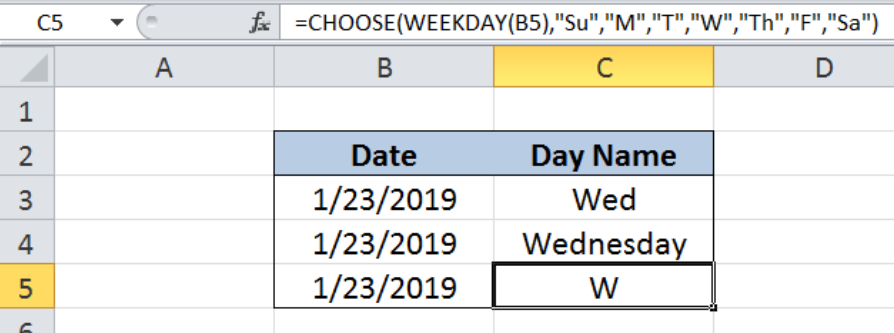Figure 6. CHOOSE and WEEKDAY functions return day name  in custom format “W”

Our formula has two parts.  First, it determines the day of the week corresponding to our date using the WEEKDAY function.  Then it chooses a value from the list based on the index number given by the WEEKDAY function.

The date “1/23/2019”, as we already know, falls on a Wednesday.  The WEEKDAY function returns the value of “4”, since by default, the value for Wednesday is 4.  We can refer back to Figure 2 above for the WEEKDAY function return_type options.

Finally, the CHOOSE function goes through the list and returns the fourth value, which is “W”, as shown in Figure 6.

## Display the day name only

Any date in Excel can be formatted to show the day name by simply changing the format.  This example will show us how to customize the display of a date to show only the day name.  Let us follow these steps:

Step 1.  Select cell C3

Step 2.  Press Ctrl + 1 to show the Format Cells dialog box

Step 3.  Select the Custom category

Step 4.  Enter “ddd” into the Type: box

Step 5.  Press Enter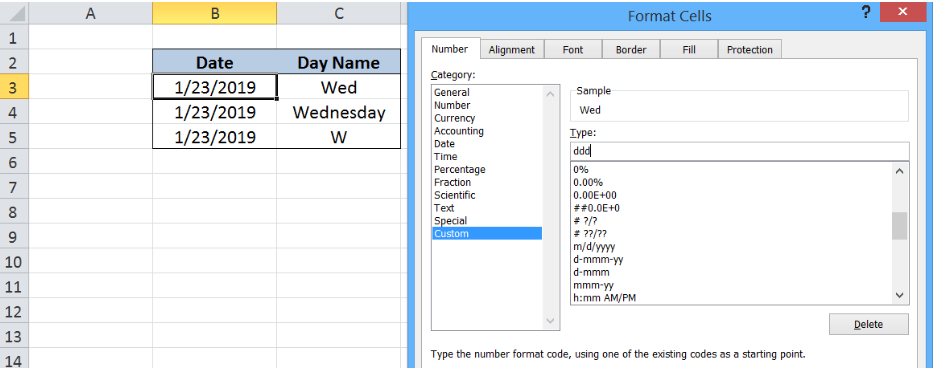Figure 7. Display day name only using the custom format”ddd”

The resulting display in cell B3 is “Wed”, an abbreviation for Wednesday and follows the format “ddd”.  Note that customizing the format doesn’t change the value of the cell. As shown below, the value of cell B3 remains the same, “1/23/2019”.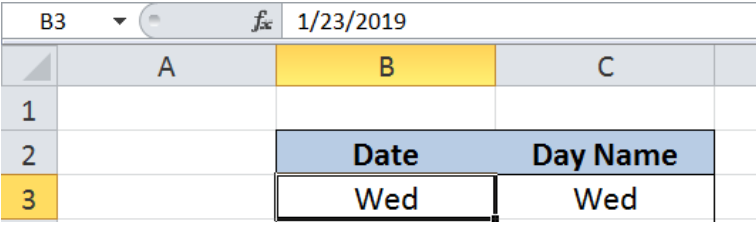Figure 8. Date displayed in custom format”ddd”

In the same manner, we can format cell B4 and enter the custom format type “dddd”.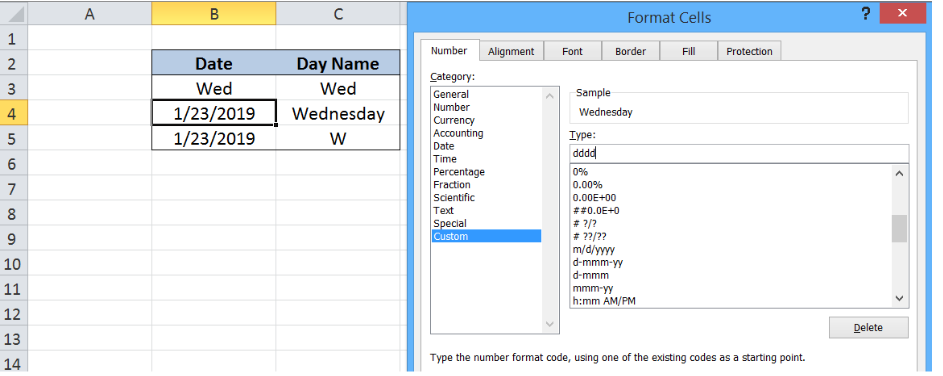Figure 9. Display day name only using the custom format”dddd”

As a result, cell B4 displays the full day name of “Wednesday”, without changing the value of the cell.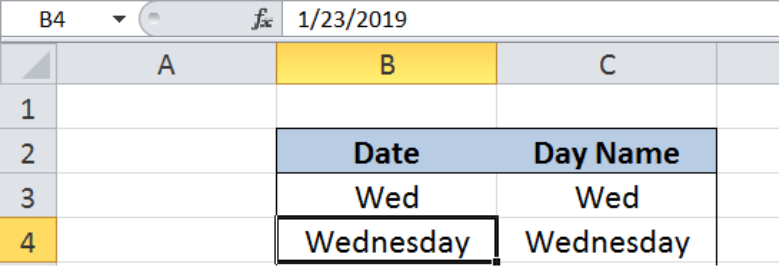Figure 10. Date displayed in custom format”dddd”

Most of the time, the problem you will need to solve will be more complex than a simple application of a formula or function. If you want to save hours of research and frustration, try our live Excelchat service! Our Excel Experts are available 24/7 to answer any Excel question you may have. We guarantee a connection within 30 seconds and a customized solution within 20 minutes.

### Did this post not answer your question? Get a solution from connecting with the expert.Another blog reader asked this question today on Excelchat:## Subscribe to Excelchat.coAnother blog reader asked this question today on Excelchat: